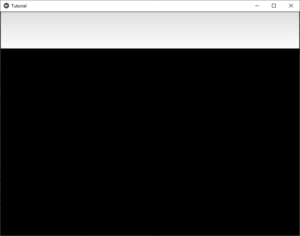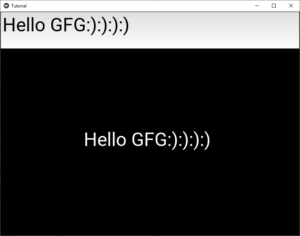GFG App
Open AppBrowser
Continue

# Python | Textinput widget in kivy

Kivy is a platform-independent GUI tool in Python. As it can be run on Android, IOS, linux and Windows etc. It is basically used to develop the Android application, but it does not mean that it can not be used on Desktops applications.

### TextInput:

The TextInput widget provides a box for editable plain text. Unicode, multiline, cursor navigation, selection and clipboard features are supported.

To create a multiline TextInput (the ‘enter’ key adds a new line).
To create a singleline TextInput, set the TextInput.multiline property to False.

`TextInput(text='Hello world', multiline=False)`

To work with Textinput you have to import it by the command –

from kivy.uix.textinput import TextInput

```Basic Approach:
1) import kivy
2) import kivyApp
3) import Label
4) import Scatter
5) import Floatlayout
6) import Textinput
7) import BoxLayout
8) Set minimum version(optional)
9) create App class
10) return Layout/widget/Class(according to requirement)
11) Run an instance of the class
```

Now the implementation of the Approach:

 `# Program to Show how to use textinput (UX widget) in kivy  ` ` `  `# import kivy module     ` `import` `kivy   ` `      `  `# base Class of your App inherits from the App class.     ` `# app:always refers to the instance of your application    ` `from` `kivy.app ``import` `App  ` `    `  `# this restrict the kivy version i.e   ` `# below this kivy version you cannot   ` `# use the app or software   ` `kivy.require(``'1.9.0'``)  ` `   `  `# The Label widget is for rendering text.   ` `from` `kivy.uix.label ``import` `Label  ` `   `  `# module consist the floatlayout   ` `# to work with FloatLayout first   ` `# you have to import it   ` `from` `kivy.uix.floatlayout ``import` `FloatLayout  ` ` `  `# Scatter is used to build interactive ` `# widgets that can be translated, ` `# rotated and scaled with two or more ` `# fingers on a multitouch system. ` `from` `kivy.uix.scatter ``import` `Scatter ` ` `  `# The TextInput widget provides a ` `# box for editable plain text ` `from` `kivy.uix.textinput ``import` `TextInput ` ` `  `# BoxLayout arranges widgets in either ` `# in vertical fashion that ` `# is one on top of another or in ` `# horizontal fashion that is one after another. ` `from` `kivy.uix.boxlayout ``import` `BoxLayout ` ` `  `# Create the App class ` `class` `TutorialApp(App): ` `     `  `    ``def` `build(``self``): ` ` `  `        ``b ``=` `BoxLayout(orientation ``=``'vertical'``) ` ` `  `        ``# Adding the text input ` `        ``t ``=` `TextInput(font_size ``=` `50``, ` `                      ``size_hint_y ``=` `None``, ` `                      ``height ``=` `100``) ` `         `  `        ``f ``=` `FloatLayout() ` ` `  `        ``# By this you are able to move the ` `        ``# Text on the screen to anywhere you want ` `        ``s ``=` `Scatter() ` ` `  `        ``l ``=` `Label(text ``=``"Hello !"``, ` `                  ``font_size ``=` `50``) ` ` `  `        ``f.add_widget(s) ` `        ``s.add_widget(l) ` ` `  `        ``b.add_widget(t) ` `        ``b.add_widget(f) ` ` `  `        ``# Binding it with the label ` `        ``t.bind(text ``=` `l.setter(``'text'``)) ` ` `  `         `  `        ``return` `b ` ` `  `# Run the App ` `if` `__name__ ``=``=` `"__main__"``: ` `    ``TutorialApp().run() `

Output:After some input –My Personal Notes arrow_drop_up